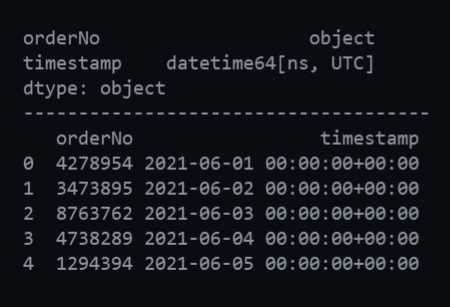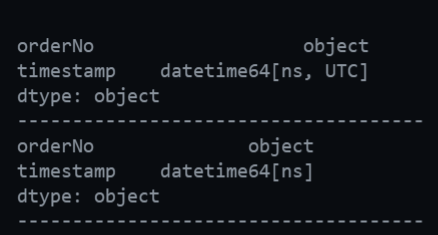Open in App
Not now

# How to remove timezone from a Timestamp column in a Pandas Dataframe

• Last Updated : 10 Sep, 2021

The world is divided into 24 timezones. We all know that different timezones are required as the entire globe is not lit at the same time. While for many instances we might not require timezones especially in cases where the data resides on a common server present at some location or even our local systems. In this article, we are going to see how to remove timezone from a Timestamp column in a pandas dataframe.

Creating dataframe for demonstration:

## Python

 `import` `pandas as pd``from` `datetime ``import` `datetime, timezone` `# CREATE THE PANDAS DATAFRAME``# WITH TIMESTAMP COLUMN``df ``=` `pd.DataFrame({``    ``"orderNo"``: [``        ``"4278954"``,``        ``"3473895"``,``        ``"8763762"``,``        ``"4738289"``,``        ``"1294394"``    ``],``    ``"timestamp"``: [``        ``datetime.strptime(``"2021-06-01"``,``                          ``"%Y-%m-%d"``).replace(tzinfo``=``timezone.utc),``        ``datetime.strptime(``"2021-06-02"``,``                          ``"%Y-%m-%d"``).replace(tzinfo``=``timezone.utc),``        ``datetime.strptime(``"2021-06-03"``,``                          ``"%Y-%m-%d"``).replace(tzinfo``=``timezone.utc),``        ``datetime.strptime(``"2021-06-04"``,``                          ``"%Y-%m-%d"``).replace(tzinfo``=``timezone.utc),``        ``datetime.strptime(``"2021-06-05"``,``                          ``"%Y-%m-%d"``).replace(tzinfo``=``timezone.utc)``    ``]``})` `# PRINT THE DATATYPES OF``# EACH COLUMN OF DATAFRAME``print``(df.dtypes)` `# VIEW THE DATAFRAME``print``(df)`

Output:Output for Code Block 1

The first part of the output tells us a timestamp column is a DateTime object. The UTC in squared brackets denotes that the timezone information is included which is actually UTC timestamp. It is because we have provided timezone as UTC.

## Method 1: Using datetime.replace() method

Datetime.replace() function is used to replace the contents of the DateTime object with the given parameters.

Syntax: Datetime_object.replace(tzinfo)

Parameters:

• tzinfo: New time zone info.

Returns: It returns the modified datetime object

Now, we will create a function to remove the timezone using the datetime module. The function will be applied to each record in the timestamp column.

## Python

 `import` `pandas as pd``from` `datetime ``import` `datetime, timezone` `# CREATE THE DATAFRAME``df ``=` `pd.DataFrame({``    ``"orderNo"``: [``        ``"4278954"``,``        ``"3473895"``,``        ``"8763762"``,``        ``"4738289"``,``        ``"1294394"``    ``],``    ``"timestamp"``: [``        ``datetime.strptime(``"2021-06-01"``,``                          ``"%Y-%m-%d"``).replace(tzinfo``=``timezone.utc),``        ``datetime.strptime(``"2021-06-02"``,``                          ``"%Y-%m-%d"``).replace(tzinfo``=``timezone.utc),``        ``datetime.strptime(``"2021-06-03"``,``                          ``"%Y-%m-%d"``).replace(tzinfo``=``timezone.utc),``        ``datetime.strptime(``"2021-06-04"``,``                          ``"%Y-%m-%d"``).replace(tzinfo``=``timezone.utc),``        ``datetime.strptime(``"2021-06-05"``,``                          ``"%Y-%m-%d"``).replace(tzinfo``=``timezone.utc)``    ``]``})` `# PRINT THE DATATYPE OF``# EACH COLUMN BEFORE MANIPULATION``print``(df.dtypes)` `# FUNCTION TO REMOVE TIMEZONE``def` `remove_timezone(dt):``  ` `    ``# HERE `dt` is a python datetime``    ``# object that used .replace() method``    ``return` `dt.replace(tzinfo``=``None``)` `# APPLY THE ABOVE FUNCTION TO``# REMOVE THE TIMEZONE INFORMATION``# FROM EACH RECORD OF TIMESTAMP COLUMN IN DATAFRAME``df[``'timestamp'``] ``=` `df[``'timestamp'``].``apply``(remove_timezone)` `# PRINT THE DATATYPE OF``# EACH COLUMN AFTER MANIPULATION``print``(df.dtypes)`

Output:Output for Code Block 2

In the output, we can see that before the manipulation of the timezone, the DateTime column i.e. the timestamp” column had the UTC timezone information. After applying the remove_timezone function on each record of the dataframe’s timestamp column, we do not see any UTC information present in the dataframe. The timestamp column in the dataframe has python datetime objects as its values. So when each of these values passes through the in remove_timezone() function it makes use of the replace() method of the Python datetime module.

## Method 2: Using Pandas

We can achieve the same without making use of the DateTime module. Let us see how –

## Python

 `import` `pandas as pd``from` `datetime ``import` `datetime, timezone` `# CREATE THE DATAFRAME``df ``=` `pd.DataFrame({``    ``"orderNo"``: [``        ``"4278954"``,``        ``"3473895"``,``        ``"8763762"``,``        ``"4738289"``,``        ``"1294394"``    ``],``    ``"timestamp"``: [``        ``datetime.strptime(``            ``"2021-06-01"``, ``"%Y-%m-%d"``).replace(tzinfo``=``timezone.utc),``        ``datetime.strptime(``            ``"2021-06-02"``, ``"%Y-%m-%d"``).replace(tzinfo``=``timezone.utc),``        ``datetime.strptime(``            ``"2021-06-03"``, ``"%Y-%m-%d"``).replace(tzinfo``=``timezone.utc),``        ``datetime.strptime(``            ``"2021-06-04"``, ``"%Y-%m-%d"``).replace(tzinfo``=``timezone.utc),``        ``datetime.strptime(``            ``"2021-06-05"``, ``"%Y-%m-%d"``).replace(tzinfo``=``timezone.utc)``    ``]``})` `# PRINT THE DATATYPE OF EACH COLUMN BEFORE``# MANIPULATION``print``(df.dtypes)` `# REMOVING THE TIMEZONE INFORMATION``df[``'timestamp'``] ``=` `df[``'timestamp'``].dt.tz_localize(``None``)` `# PRINT THE DATATYPE OF EACH COLUMN AFTER``# MANIPULATION``print``(df.dtypes)`

Output:Output for Code Block 3

In the above example, we can see that the dt.tz_localize(None) method can be applied to the dataframe column to remove the timezone information. The output similar to the above example reflects that after manipulation, the UTC timezone information is no longer present in the timestamp column.

My Personal Notes arrow_drop_up